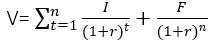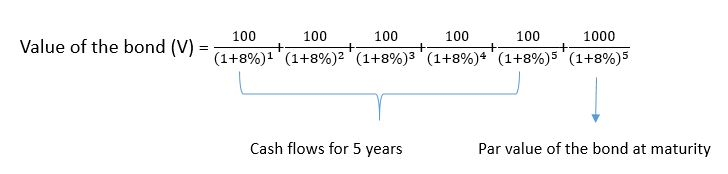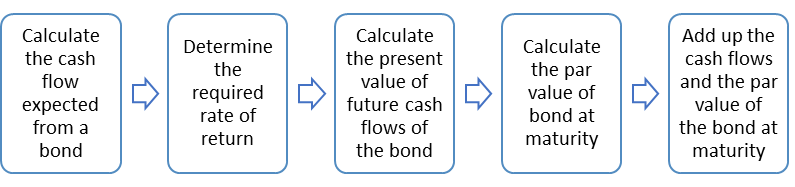# Bond Pricing

Bond Pricing/bond valuation is a method of calculating the fair price or value of a bond. The price of a bond is calculated by finding out the present values of future cash flows and discounting them at an appropriate discount factor.

## Understanding Bond Terminology

Before we learn how to determine the price of a bond, let us understand some bond terminology.

A bond is a note issued by governments or corporates that promises its buyer a specified amount of money after a fixed duration (maturity). It also agrees to pay a certain amount as interest to the buyer until the maturity. This interest rate is called the coupon rate. Hence, the investor receives a total cash flow of interest paid every year until maturity plus the value of the bond.

### Example:

A 5-year bond of \$1000 face value at a 5% coupon rate means, a firm has borrowed \$1000 from the buyer of a bond and promises him (the buyer) to repay the \$1000 after five years plus an interest of 5% paid every year. \$1000 is the par value or face value of the bond, 5 years is the maturity period, 5% is the coupon or interest rate.

So, the total amount the buyer gets is,

1st Year: \$50

2nd Year: \$50

3rd Year: \$50

4th Year: \$50

5th Year: \$50 + \$1000

Total amount: (5*\$50) + \$1000 = \$1250

Hence, the profit made by the buyer of the bond is \$250.

## Need for Bond Pricing

If an investor buys a security, he ensures he gets the best returns out of it. Hence, he always compares among other securities available in the market to choose the best one to buy. So, an investor decides on buying a particular bond, if it offers better returns compared to its peers available in the market, given the risk associated with them is the same.

However, bonds usually do not trade at par value in the open market. They either trade at a discount or at a premium depending on the interest rate environment prevailing in the market. Here arises the need to calculate the actual value of the bond (called as fair value or intrinsic value) to determine if it is a good buy or not with respect to the current interest rate offered in the market. If the interest rates in the market are always constant, there is no question of valuing a bond.

The main objective of valuing a bond is to compare if, the returns offered by the bond (known as bond yield) an investor wishes to buy is greater than or equal to the risk free interest rate (interest rate offered by no risk securities Example: 3-month treasury bills in the US) present in the market. The

## How to Find the Price a Bond?

As mentioned above, the right technique to value a bond is to find out the present value of the future cash flows of the bond. Cash flows from the bond are nothing but the coupon payments made every year (or quarter or semi-annually). The final bond price is the sum of all the coupon payments of each year until maturity plus the face value of the bond (as shown in example 1).

Cash flows of each year are calculated by finding an appropriate discount factor and discounting the present value of the coupon payments using this rate. The discount factor is determined as the interest rate, which an investor will get if he/she holds the bond till maturity. This is called yield to maturity (YTM).

The formula for calculating the value of a bond (V) isI = annual interest payable on the bond

F= Par value of the bond (repayable at maturity)

r = discount factor or required rate of return

n= maturity of the bond

### How to Determine the Discount Rate?

YTM is the return an investor gets if he holds the bond until maturity. The discount rate is the interest rate that the investor wishes to get as a return (in terms of interest %) with respect to the current interest rates prevailing in the market.

After plugging in all the values in the above formula, one can calculate the price of a bond.

The following example helps to understand this concept better.

#### Example 2:

Calculate the price of a bond whose face value is \$1000, the coupon rate is 10% and will mature after 5 years. The required rate of return is 8%.

Coupon payment every year is \$1000*10% = \$100 every year for a period of 5 years.

Hence,Therefore, the value of the bond (V) = \$1079.8

The following is the summary of bond pricing:Last updated on : October 25th, 2019